# BAQ - Time Conversion

Hi,
I am trying to calculate the Actual reported time. Actual Reported Time = Clock in Time – Current Time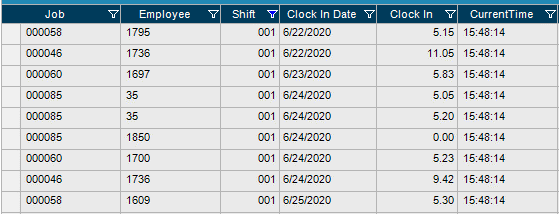It is throwing the below error while running the test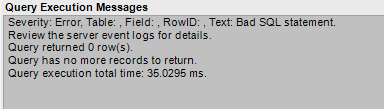Thanks
Subha

Try using the calculated field that shows the Current Time, instead of the `Constants.CurrentTime`

Calvin, I am not finding any CurrentTime option in calculated field.

What is creating this field?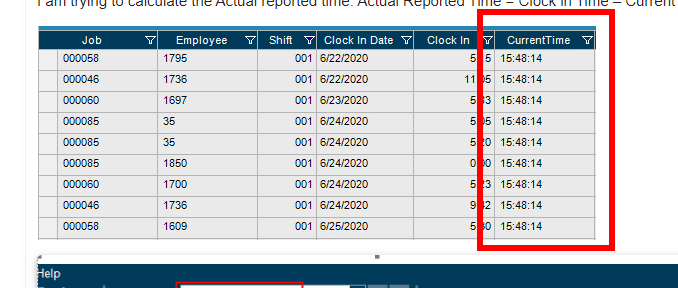I created the current time calculated field as below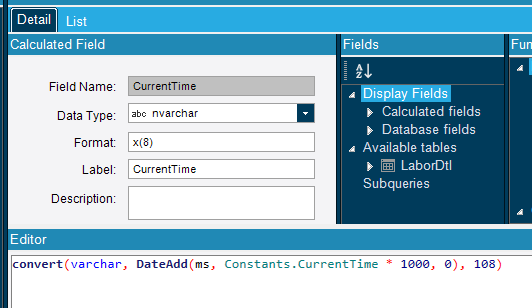Okay. I see the issue now…

the formula should have the LaborDtl.ClockinTime in the DateAdd()

something like

``````(convert(varchar, DateAdd(ms,Constants.CurrentTime * 1000, LablorDtl.ClockInTime), 108)
``````

FWIW - I haven’t checked that, as I don’t know what the data type of the ClockInTime is.

Calvin,
The problem is I am not able to do arithmetic function - subtraction in varchar datatype. how to convert the current time varchar data type to integer in the time format.
Currently we have Labordtl.ClockInTime in integer data type. I have to convert the current time to integer data type to do the subtraction function.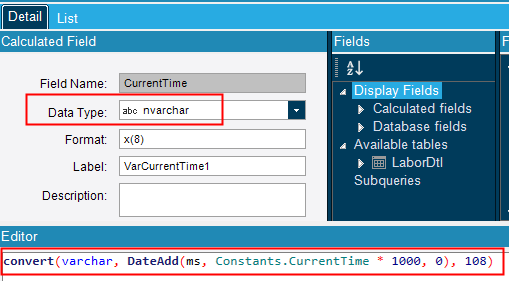I meant in the ActualReportedTime calculated field.

And look at it as two steps:

1. Calculate the new time - Using DateAdd(), you can add (or subtract) the ClockInTime from the CurrentTime. with:
`DateAdd(ms, -Constants.CurrentTime*1000, LaborDtl.ClockinTime)`
2. convert that result to a string - using convert():
`convert(varchar, [the stuff from step 1] , 108)

And FTR I would have this column be of type Time, or DateTime. That way you could use it for math against other values, or even filter on it.

# edit

I just now see that the CurrentTime is in seconds since midnight. So first you have to make a date time from Constants.Today & Constants.CurrentTime.
` DateAdd(s,Constants.CurrentTime,Constants.Today)` - That will give you the current time(and date) in a DateTime value

Next, subtract the ClockinTime (which is in hours) from the above. Do this by using the `hour` interval, and a negative sign in front of the ClockInTime

`DateAdd(hour, -LaborDtl.ClockinTime,DateAdd(s,Constants.CurrentTime,Constants.Today))`

If you really want it as a string add the `convert(varchar, .the above…, 108)

Thanks Calvin